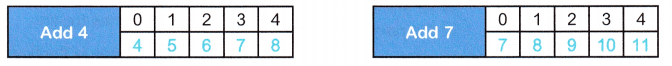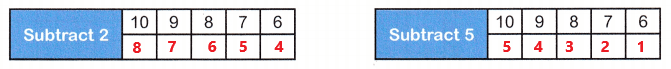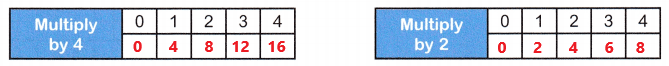# McGraw Hill Math Grade 5 Chapter 11 Lesson 6 Answer Key Creating Number Patterns

All the solutions provided in McGraw Hill Math Grade 5 Answer Key PDF Chapter 11 Lesson 6 Creating Number Patterns are as per the latest syllabus guidelines.

## McGraw-Hill Math Grade 5 Answer Key Chapter 11 Lesson 6 Creating Number Patterns

Calculate

Complete the patterns in each set of tables. Then compare each pattern and write about your comparison.

Question 1.Compare the patterns. What is their relationship?
Each number in the second pattern is 3 more than the number in the first pattern.

Question 2.Compare the patterns. What is their relationship?Explanation:
The pattern in left hand side table is 8, 7, 6, 5, 4.
The pattern in right hand side table is 5, 4, 3, 2, 1.
Each number in the second pattern is 3 less than the number in the first pattern.

Question 3.Compare the patterns. What is their relationship?